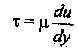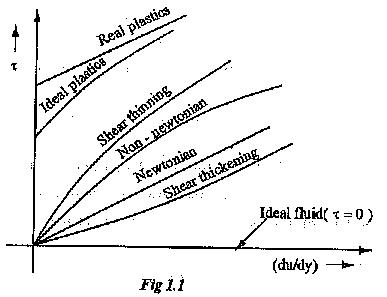## Classification of Fluids:

Classification of Fluids are as follows.

• Ideal fluids and Real or Practical fluids.
• Newtonian fluids and Non-Newtonian fluids.

### 1 .Ideal Fluids:

Ideal fluids are having following properties.

• It is incompressible.
• It has zero viscosity.
• Shear force is zero when the fluid is in motion i.e. No resistance is offered to the motion of any fluid particles.

### 2. Real or Practical Fluids:

(i) It is compressible.

(ii) They are viscous in nature.

(iii)Some resistance is always offered by the fluid when it is in motion.

(iv) Shear stress always exists in such fluids.

### 3. Newtonian Fluids:

In Newtonian fluids a linear relationship exists between the magnitudes of shear stress τ and the resulting rate of deformation (du/dy). i.e. the constant of proportionality μ does not change with the rate of deformation.Example: Water, Kerosene,

The viscosity at any given temperature and pressure is constant for a Newtonian fluid and is independent of the rate of deformation.

### 4. Non Newtonian Fluid:

In Non-Newtonian fluids, there is a non-linear relation between the magnitude of the applied shear stress and the rate of deformation. The viscosity will vary with variation in rate of deformation. They do not obey Newton’s law of viscosity.

The Non-Newtonian fluids can be further classified into five groups. They are simple Non-Newtonian, ideal plastic, shear thinning, and shear and real plastic fluids.